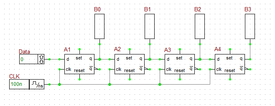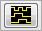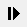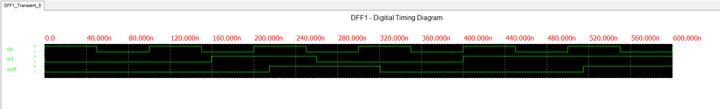# Digital Tutorial Lesson 3: Building a Shift Register Using D Flip-Flops

 Tutorial Project: Exploring Flip-Flops and Sequential Logic CircuitsObjective: In this project, the basic concepts of RF.Spice A/D are demonstrated, and a simple voltage divider is modeled and examined. Concepts/Features: Minimum Version Required: All versions

### What You Will Learn

In this tutorial lesson, first you will build SR flip-flop circuits out of logic gates. You will examine their truth table and use them to verify the operation of B2.Spice's own generic SR latch device. Then you will examine B2.Spice's D flip-flop device and will use four D flip-flops to design a 4-bit shift register. Finally, you will examine B2.Spice's JK flip-flop device and will use four JK flip-flops to design a 4-bit binary counter. You will use both the live timing diagrams and transient analysis in this tutorial lesson.

## Testing a D Flip-Flop

The following is a list of parts needed for this part of the tutorial lesson:

Part Name Part Type Part Value
In1 Digital Input 1-bit
Out1 Digital Output N/A
CLK Digital Clock Period = 100ns, Pulse Width = 50ns
A1 D-Type Flip-Flop Defaults

You will build a synchronous circuit with a digital clock and a single input as shown in the figure below. The keyboard shortcut for digital clock is Alt+C. Set the "Period" of the clock to 100ns and its "Pulse Width" to 50ns. Connect the digital input device to the "D" pin of the flip-flop and the digital output device to its "Q" pin. The D flip-flop transfers the input data at its input to its Q output on the rising edge of the clock pulse. During the rest of the clock cycle, the output remains unchanged (hold state).The D-Type Flip-Flop device of RF.Spice A/D.

To understand the operation of the flip-flop circuit, you will use the "Live Digital Timing Diagram" feature of RF.Spice A/D. As you earned in the previous tutorial lesson, set the step time to 20ns. Set the input initially to In1 = 0. Then, to activate the timing diagram, click the "Show/Hide Live Digital Timing Diagrams"button of the Schematic Toolbar. Remember that nothing will happen until you start a digital simulation. Click the "Step"button of the Main Toolbar or use the keyboard shortcut Ctrl+H to advance the simulation time one step at a time. Step the simulation time to 60ns and then change the input to In1 = 1. Keep stepping to 160ns and then change the input to In1 = 0 again. Continue stepping to 400ns and change the input to In1 = 1 once again and then proceed to 600ns. The table below summarizes the input entries:

Time In1
0ns 0
160ns 1
260ns 0
400ns 1

The timing diagram of your D flip-flop circuit is shown in the figure below. As you can see from the figure, the input first rises from 0 to 1 at t = 160ns. The flip-flop waits until the next rising edge of the clock signal at t = 200ns. The input's high state is transferred to the output Q with a propagation delay of 14ns. According to the property dialog of the D Flip-Flop device, the low-to-high propagation delay of the device is tPLH = 14ns, while its high-to-low propagation delay is tPHL = 1420ns. Therefore, Q rises from 0 to 1 at t = 214ns and stays high for the next several clock cycles. The input next falls from 1 to 0 at t = 260ns. The flip-flop waits until the next rising edge of the clock signal at t = 300ns. The input's low state is transferred to the output Q with a propagation delay of 20ns. Therefore, Q falls from 1 to 0 at t = 320ns and stays low for the next several clock cycles. The input rises again from 0 to 1 at t = 400ns and stay high for the rest of the simulation time. This time the flip-flop has to wait a full clock period until the next rising edge of the clock signal at t = 500ns. The input's high state is transferred to the output Q with a propagation delay of 14ns. Then, Q rises again from 0 to 1 at t = 514ns and stays high for the rest of the simulation time.The digital timing diagram of the single D-Type Flip-Flop circuit.

## Building a 4-Bit Shift Register Using D Flip-Flops

The following is a list of parts needed for this part of the tutorial lesson:

Part Name Part Type Part Value
Data Digital Input 1-bit
B0 - B3 Digital Output N/A
CLK Digital Clock Period = 100ns, Pulse Width = 50ns
A1 - A4 D-Type Flip-Flop Defaults

A shift register is a multi-output digital circuit that transfer the input data to its outputs sequentially at each clock cycle. Cascade the four D flip-flops as shown on the figure by connecting the Q pin of each to the D pin of the next. All four flip-flops share the same clock signal. Use the same clock settings from the previous part (Period = 100ns and Pulse Width = 50ns). Set the step time to 20ns. Enable the live digital timing diagram. Run a digital simulation and step through 1200ns. Set the value of the input "Data" to 0 and change it to 1 at t = 100ns and change it back to 0 at t = 600ns.

Your timing diagram will look like the figure below. As you can see from the figure, after the input is set high at t = 100ns for the first time, it takes the first flip-flop until the clock's next rising edge at t = 200ns to react. The first output B0 rises to 1 at t = 214ns (tPLH = 14ns). As the clock cycles proceed, the other outputs B1, B2 and B3, sequentially rise to 1 at t = 314ns, 414ns, and 514ns, respectively. All four outputs remain high for the next several clock cycles (at hold state).

The input "Data" next falls from 1 to 0 at t = 600ns. It takes the first flip-flop until the clock's next rising edge at t = 700ns to react. The first output B0 fall from to 0 at t = 720ns (tPHL = 20ns). As the clock cycles proceed, the other outputs B1, B2 and B3, sequentially fall to 0 at t = 820ns, 902ns, and 1020ns, respectively. All four outputs remain low afterwards (at hold state).The timing diagram of the 4-bit shift register circuit.